# Estimate the effect of population-wide face mask wearing

## Motivation

Masks are not perfect in blocking coronavirus. Even for the surgical masks which theoretically filter ~90% of the particles in relevant size, they might be inappropriately worn by the general public.
Considering the significant portion of the pre-symptomatic transmission, can masks with inadequate filtering efficacy help to contain the COVID19 pandemic, if applied on a population-wide scale?

## Result in three sentences

Yes. In our highly simplified model, there is an aggregated effect of population-wide mask-wearing: The effect of mask filtering efficacy and the percentage of mask-wearing population multiply, and act in a non-linear manner. Even if masks only block ~50% of the virus have the potential of stopping the pandemic, if adopted by 100% of the population.

## Model Description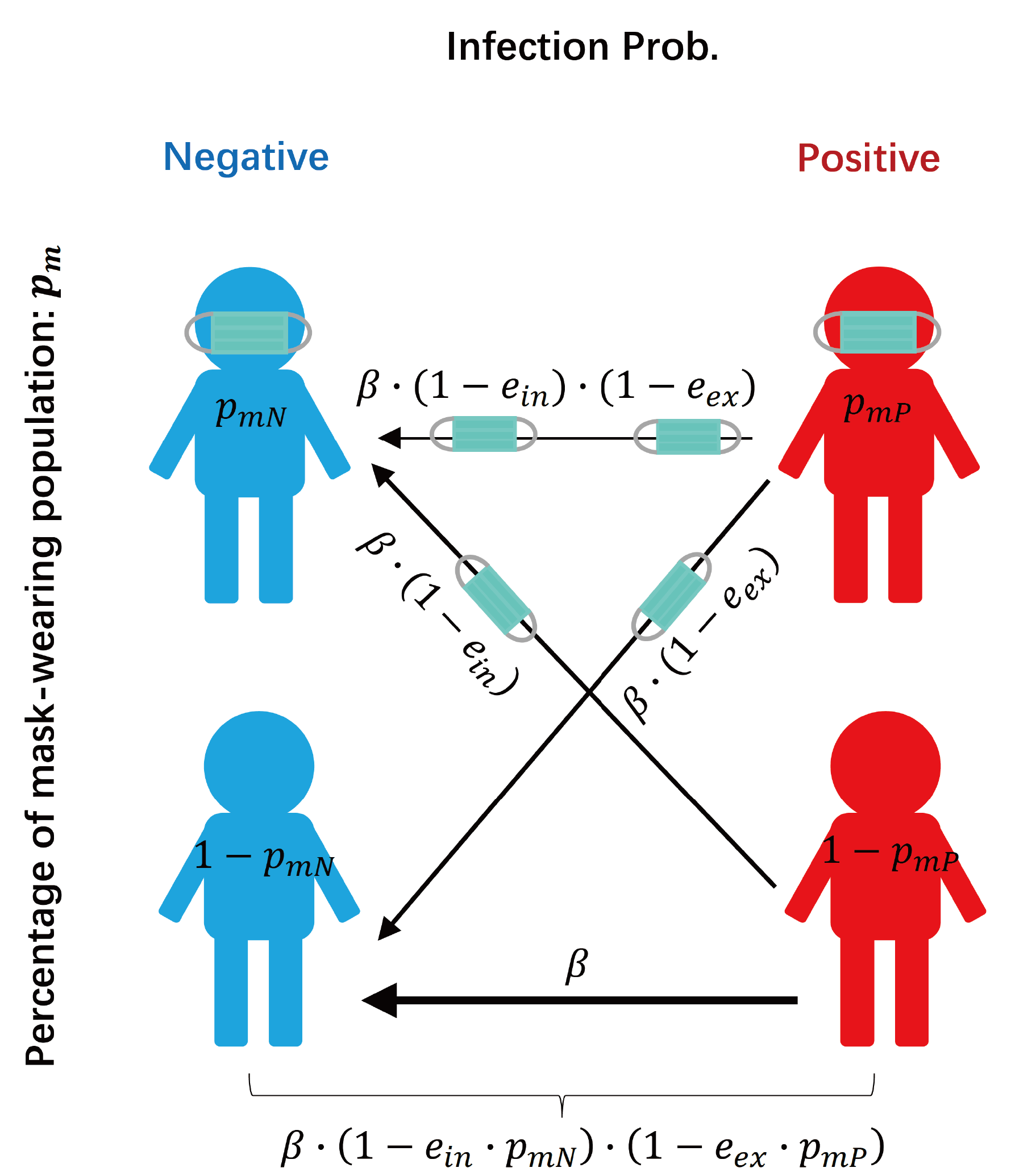Symbols:
Positive and Negative individuals are marked as P and N.
The percentage of mask-wearing population p_m: pm_P in Positive population, pm_N in negative population.
The filtering efficacy of mask e: the percentage of virus trapped by a mask, in exhaling (e_ex) from positive individual and in inhaling (e_in) by negative individual.
The percentage of virus transmitted by respiratory routes f: Not all virus are transmitted by respiratory routes and can be stopped by masks. On average, between positive and negative invidividuals, the fraction of virus transmitted by respiratory routes is f_res, the fraction of virus transmitte by contact routes is f_con. Contact-based transmission is more common within family. Usually, We set f_res + f_con=1, when there is no other intervension;
The relative probabilty of infection i(v) given the relative viral load v: The amount of virus received by a healthy individual may not map linearly to the probability of being infected. We set the function I(V) to be the probability of being infected given the viral load V. As we are interested in comparing the relative decrease of the infectivity, we set i(v) to be the relative probability of getting infected when viral load decrases to v percent of its regular value, normalized by the transmission probability with full viral load V_full when two individuals are both without masks. i(v)=I(v*V_full)/I(V_full); By the simplest assumption, i(v) it is linear. But more reasonably, it may take the sigmoid shape. But always, i(0)=0 and i(1)=1;

## Analytical Solution for the Reduction of Transmission Rate

In a well-mixed model, when a negative and a positive individual meet, there are 4 types of mask-wearing situation:
1. N has mask, P has mask (probability: pm_P*pm_N), with the relative infection probability i( f_con + f_res*(1-e_ex)*(1-e_in) );
2. N does not have mask, P has mask (probability: pm_P*(1-pm_N) ), with the relative infection probability i( f_con + f_res*(1-e_in) );
3. N has mask, P does not have mask (probability: (1-pm_P)*pm_N), with the relative infection probability i( f_con + f_res*(1-e_ex) );
4. N does not have mask, P does not have mask (probability: (1-pm_P)*(1-pm_N)), with the relative infection probability i( f_con+ f_res );
Assume r is the factor of reduction on the transmission rate, after population-wide masks wearing. We name it "mask reduction factor". The value of r is calculated as:
r =pm_P*pm_N*i( f_con + f_res*(1-e_ex)*(1-e_in) ) + pm_P*(1-pm_N) * i( f_con + f_res*(1-e_in) ) + (1-pm_P)*pm_N * i( f_con + f_res*(1-e_ex) ) + (1-pm_P)*(1-pm_N)*i( f_con+ f_res )
For R0 = 3.68, r needs to be as small as 0.27.
The values of e_ex, e_in, and f_res and f_con, and the form of i(v), all influence r, the effect of population wide-mask wearing.
In the code below, one can try to assign one set of values for e_ex, e_in, and f_res and f_con, and the form of i, to check how muchthe transmission rate can be reduced.
% In the form of functions, r is:
rvalue=@(e_ex,e_in,f_res,f_con,pm_P,pm_N,i)pm_P.*pm_N.*i( f_con + f_res.*(1-e_ex).*(1-e_in) ) + pm_P.*(1-pm_N) .* i( f_con + f_res.*(1-e_in) ) + (1-pm_P).*pm_N .* i( f_con + f_res.*(1-e_ex) ) + (1-pm_P).*(1-pm_N).*i( f_con+ f_res );
%%%%%%%%%
% Customizable input values
R0=3.68; % initial R0 without
e_ex=0.5; % 50% of the virus is trapped in exhalation
e_in=0.3; % 50% of the virus is trapped in inhalation
pm_P=0.9; % 90% of the COVID19-positive individuals wear masks
pm_N=0.5; % 50% of health individuals wear masks
f_res=0.7; % 70% of the transmission can be attributed to respiratory routes
ifun=@(v)v; % the probability of getting sick is linear with the viral load
%%%%%%%%%
f_con=1-f_res; % default: the rest of the transmission is by contact and cannot be reduced by masks
fprintf('r =\n')
r =
r=rvalue(e_ex,e_in,f_res,f_con,pm_P,pm_N, ifun)
r = 0.6833
fprintf('R reduces from %f to %f\n',R0, R0*r)
R reduces from 3.680000 to 2.514360

## Simplified Solutions under Different Assumptions

Even in this simplified model, there are still six factors influence the effect of population wide mask-wearing: e_ex,e_in,f_res,pm_P,pm_N, ifun().
We can make more assumptions to gain more intuitions on the effect of masks.
We can take different assumptions to make the form of r simpler:
syms e_ex e_in e pm_P pm_N pm f_res f_con
i=@(v)v;
r =pm_P*pm_N*i( f_con + f_res*(1-e_ex)*(1-e_in) ) + pm_P*(1-pm_N) * i( f_con + f_res*(1-e_in) ) + (1-pm_P)*pm_N * i( f_con + f_res*(1-e_ex) ) + (1-pm_P)*(1-pm_N)*i( f_con+ f_res )
r =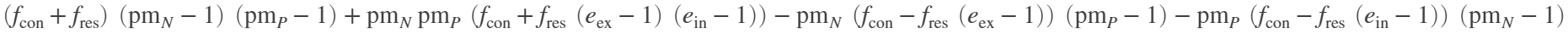fprintf('\nMask reduction factor with i in linear form, and f_con+f_res=1:\n')
Mask reduction factor with i in linear form, and f_con+f_res=1:
simplify(subs(expand(r),f_con,1-f_res),'IgnoreAnalyticConstraints', true)
ans =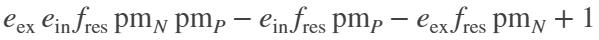fprintf('\nMask reduction factor when e_ex=e_in=e, and f_con+f_res=1:\n')
Mask reduction factor when e_ex=e_in=e, and f_con+f_res=1:
simplify(subs(expand(r),[e_ex,e_in,f_con],[e,e,1-f_res]),'IgnoreAnalyticConstraints', true)
ans =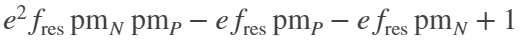fprintf('\nMask reduction factor when pm_P=pm_N=pm, and f_con+f_res=1:\n')
Mask reduction factor when pm_P=pm_N=pm, and f_con+f_res=1:
simplify(subs(expand(r),[pm_P, pm_N,f_con],[pm,pm,1-f_res]),'IgnoreAnalyticConstraints', true)
ans =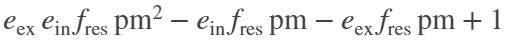fprintf('\nMask reduction factor when pm_P=pm_N=pm, and e_ex=e_in=e,and f_con+f_res=1:\n')
Mask reduction factor when pm_P=pm_N=pm, and e_ex=e_in=e,and f_con+f_res=1:
simplify(subs(expand(r),[pm_P, pm_N, e_ex,e_in,f_con],[pm,pm,e,e,1-f_res]),'IgnoreAnalyticConstraints', true)
ans =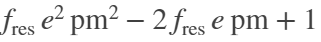fprintf('\nMask reduction factor when pm_P=pm_N=pm, and e_ex=e_in=e, and f_con=0:\n')
Mask reduction factor when pm_P=pm_N=pm, and e_ex=e_in=e, and f_con=0:
simplify(subs(expand(r),[pm_P, pm_N, e_ex,e_in,f_con,f_res],[pm,pm,e,e,0,1]),'IgnoreAnalyticConstraints', true)
ans =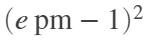## Visualization

Under the last and the most simplified assumption (e_in=e_ex=e; pm_P=pm_N=pm, f_con=0), r=(e*pm-1)^2,. It suggests the effect of mask wearing to be non-linear: the more people wear masks, the more effective this measurement would be. If 100% of the population wears masks, e only needs to be 0.5 to fully contain the virus even for a R0 = 3.68.
%%%%%%%%% simplified assumptions
f_con=0;
f_res=1;
ifun=@(v)v; % the probability of getting sick is linear with the viral load
R0=3.86; % it ranges from 2 to 6 from different researches
%%%%%%%%%%
pointnum=50; % number of points
pmlist=linspace(0,1,pointnum);
elist=linspace(0,1,pointnum);
pm_matrix=zeros(pointnum);
e_matrix=zeros(pointnum);
r_matrix=zeros(pointnum);
for i=1:pointnum
for j=1:pointnum
pm=pmlist(i);
e=elist(j);
pm_matrix(i,j)=pm;
e_matrix(i,j)=e;
r_matrix(i,j)=rvalue(e,e,f_res,f_con,pm,pm, ifun);
end
end
figure;
ph=pcolor(100*e_matrix,100*pm_matrix,R0*r_matrix);
ph.LineStyle='none';
hold on;
contour(100*e_matrix,100*pm_matrix,R0*r_matrix,[1,2,3],'k','ShowText','on');
ch=colorbar;
axis square
set(gca,'fontsize',18);
xlabel({'Efficacy','How well the mask blocks the virus'})
ylabel(ch,'Re under intervention')
title({sprintf('R0 = %0.2f',R0),'Mask reduction factor (1-e*pm)^2'})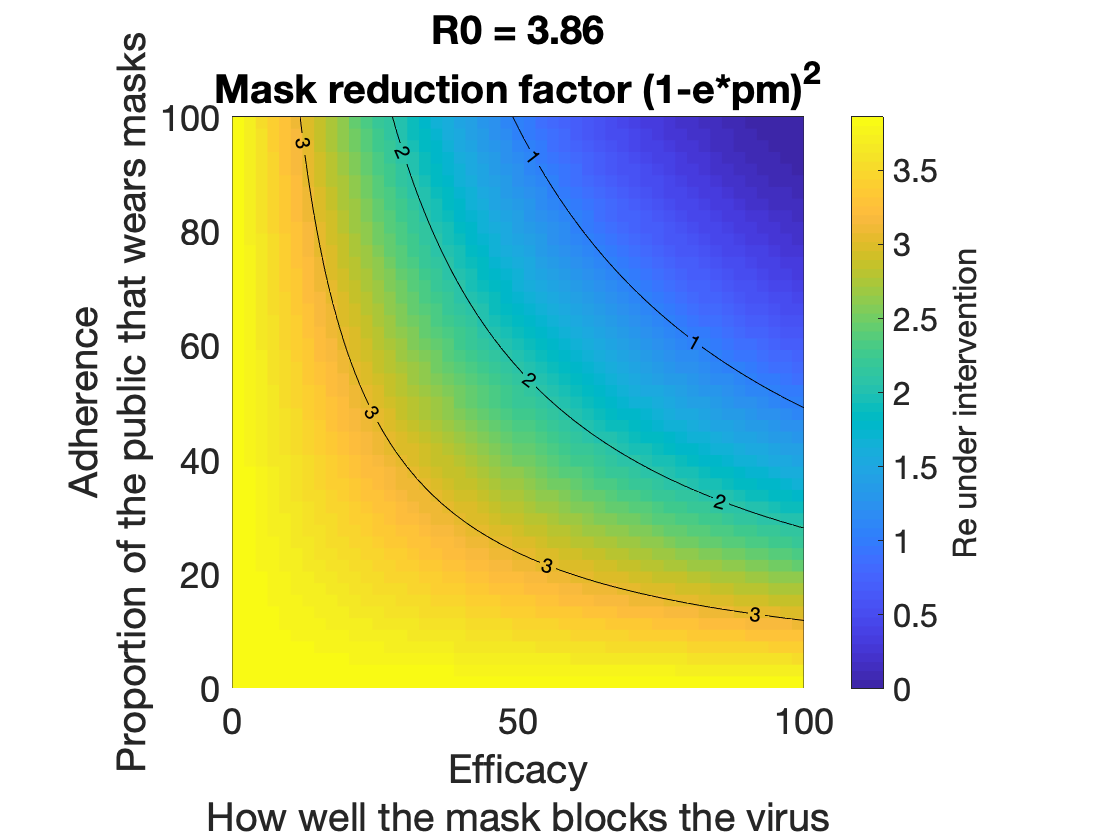## The Effect of f_res

Not all transmission can be attributed to respiration. For the contact-based transmission, masks does not help much. When we consider different values of f_res (still under the simplification that e_in=e_ex=e; and pm_P=pm_N=pm, ifun is linear), The relationship between effective transmission Re and the mask wearing population becomes:
figure;
hold on;
pointnum=20;
pmlist=linspace(0,1,pointnum);
elist=[0.3,0.5,0.7,0.9];
legendstr=[];
f_res=0.5
f_res = 0.5000
for i=1:length(elist)
e=elist(i);
R0fun=@(pm)R0*rvalue(e,e,f_res,1-f_res,pm,pm,ifun);
plot(100*pmlist,R0fun(pmlist),'linewidth',1.5)
legendstr{i}=['Efficacy = ',num2str(100*e),'%'];
end
ylim([1,R0])
box on
set(gca,'fontsize',18)
legend(legendstr)
ylabel('Re under intervention')
title(sprintf('f_{res} = %0.1f',f_res))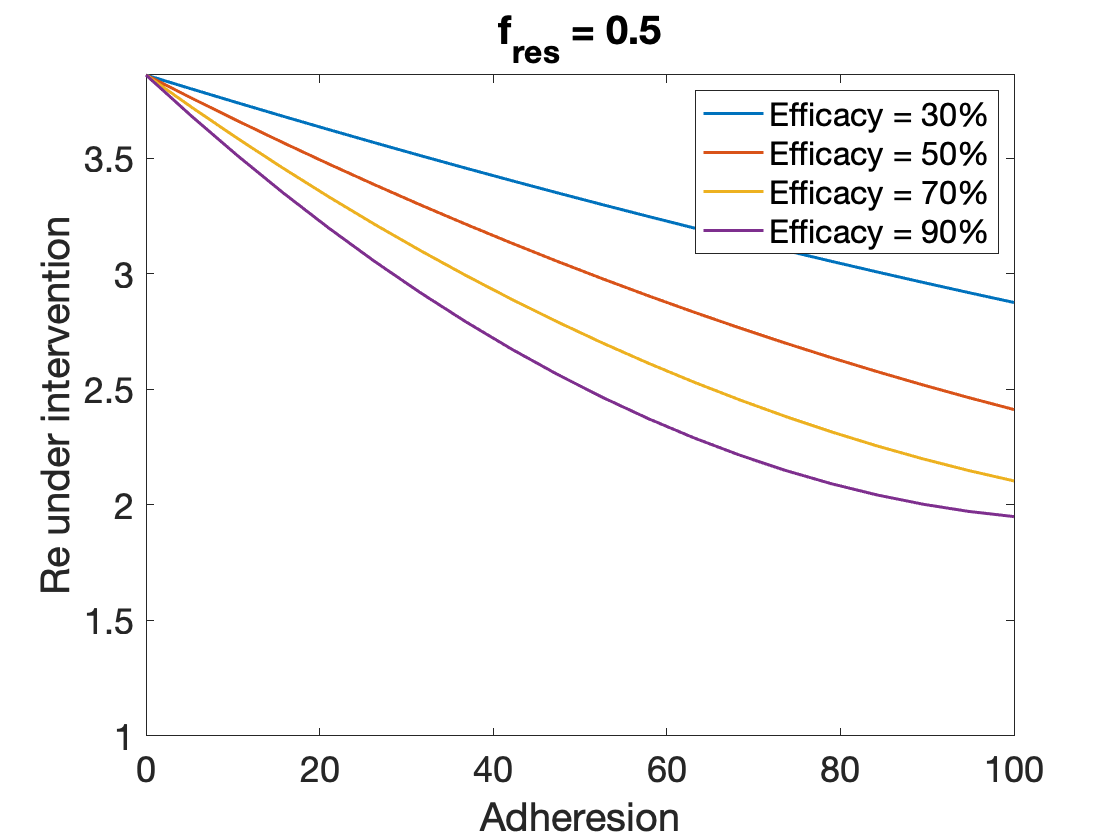## The Effect of non-linear ifun()

The relationshiop between the amount of virus being transmitted and the probability of getting sick might not be linear, with the non-linearity of ifun() being considered (still under the simplification that e_in=e_ex=e; and pm_P=pm_N=pm, f_res=1), The relationship between effective transmission Re and the mask wearing population becomes:
figure;
hold on;
pointnum=20;
pmlist=linspace(0,1,pointnum);
elist=[0.3,0.5,0.7,0.9];
legendstr=[];
f_res=1;
n=5;
ifun=@(v)1/(1+(0.5/v)^n)/(1/(1+(0.5)^n));
for i=1:length(elist)
e=elist(i);
R0fun=@(pm)R0*rvalue(e,e,f_res,1-f_res,pm,pm,ifun);
plot(100*pmlist,R0fun(pmlist),'linewidth',1.5)
legendstr{i}=['Efficacy = ',num2str(100*e),'%'];
end
ylim([1,R0])
box on
set(gca,'fontsize',18)
legend(legendstr)